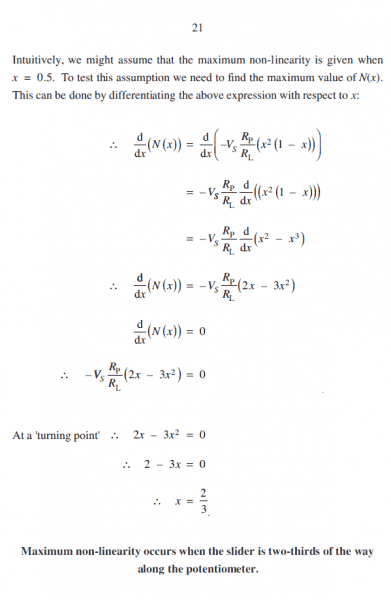# Wirewound Potentiometer question

ilovescience85

## Homework Statement

A Wirewound potentiometer having 300 turns is to be used in the following system (see atached question file)

## Homework Equations

See attached coursework file

## The Attempt at a Solution

Unsure where to begin. I have converted the circuit in the attached file to a Thévenin equivalent circuit but I'm unsure what figures to use for my Rt when the tapper is at 50%. Would i be right in saying that for the 50% tapper that x would equal 0.5 so (1-x)Rp would be (1-0.5)3kΩ and xRp would be 0.5x3kΩ both equalling 1.5kΩ.

Its the equations for working out the non-linearity which you can see on pages 19-21 of the attached learning material that i cannot follow. Any help would be greatly appreciated.

I have attache two files, one is the question and the other is the learning material given regarding the question.

#### Attachments

59.3 KB · Views: 248
•Question 3.PNG
53.1 KB · Views: 571

Homework Helper
Gold Member
What are you planning to do with the 0.5 kΩ?

ilovescience85
I am unsure on which equation I should use, the equations on pages 19 & 20 are obviously the route I should be taking but I'm unsure of where to begin. I attempted to use Vactual = Vt x RL / RL + RT and I got a figure of 5v but this is obviously the linear figure. So do I simply take x as 0.5 and input it to the following equation N(x)=-Vs x (x2(1-x)/ RL/Rt + x(1-x) along with the figures for Vs, RL and Rt?

Homework Helper
Gold Member
You have a single, adjustable resistance between the tap and the 20 V source that is in series with two resistances that are in parallel. What is the resistance of the two resistances that are in parallel? What is the total resistance when that is in series with the single resistance?

ilovescience85
Parallel resistance is Rt= R2xRL/R2+RL? Series resistance Rt= R1+R2 so parallel Rt= 1.5x0.5/1.5+0.5=0.375 series =1.5+0.375=1.875
I=v\r 20/1.875= 10.67 Vout = Ixr = 10.67 x 0.375= 4.00125v @ 50%tap? Am I miles off?

Homework Helper
Gold Member
Looks pretty good. A note on your notation and units: be careful when you're "dropping" the "k" on kΩ and switching to mA rather than A for current; if you go ahead and write those units in, you won't find yourself in trouble with more involved problems where it's easy to lose (or gain) three orders of magnitude in your answers.

ilovescience85
Ah ok yeah i'll keep a track on my notation. So my next question is when would all the equations within the learning material be required to be used?

Homework Helper
Gold Member
For some silly reason my browser doesn't want to open pdfs when I link to them secondhand, so I couldn't begin to guess

ilovescience85See if these pictures work? this is what is in the learning material when it talks about the loading effect on a potentiometer.

#### Attachments

59.3 KB · Views: 236
Homework Helper
Gold Member
Readable. You understand the equations? And just want to know when to use them? Or, what use you can make of them? Or, why the great religious ritual to demonstrate that a passive voltage divider is non-linear?

ilovescience85
My differentation is great but i understand the flow of the equations and that all in all it is simply proving that the voltage divider is no linear and it's max non-linearity is when the potentiometer is 2/3 of the way along it's slider. I think i need to be at a better level of differentation before i can use them, yeah i find there is a lot that within this learning material. Also not a lot of guidance when i comes to wether its purely to show working/understanding or if it will be required for calculation purposes.

I've had to create a graph of tapper % position v output voltage, it's now asking me to calculate the maximum error as a percentage of he true value. Can i simply take the 2/3 position and calucate the error there with respect to true value?

Homework Helper
Gold Member
Without doing the math, "that will be left as an exercise for the reader," (how many times have you had that in your face?) it's not obvious that maximum non-linearity is coincident with maximum error. You'll want to set up ideal Vout for no load (infinite resistance across divider output), x or (1-x) times 20 V minus Vout for the 500 ohm load over ideal Vout, and differentiate that with respect to x, looking for its maximum.

ilovescience85
So taking the figure i have the maximum error comes out at 50% so i understand that the max error does not coincide with max non-linearity. See attached image. I also understand that there is a point between 50% and 60% tapper position wherer the error will be it's max but I'm unsure how to find the maximum like you've explained above.